# Informed Search Algorithms#

Informed search algorithms differ from blind search algorithms in the sense that the goal state is already known. Additionally, the algorithms use knowledge during the search in order to guide the search. This knowledge can take the form of distance to target, or incurred costs.

## Minimum Spanning Tree#

Given a spanning tree $$T$$ in a graph $$G$$ with a weight $$w$$ and edges $$E$$, the weight $$w$$ of $$T$$ is the sum of weights of its edges. If $$T$$ has the minimum weight over all spanning trees of $$G$$, we call $$T$$ a minimum spanning tree.

### Disjoint Sets#

Before going any further and introducing the algorithm that generates the minimum spanning $$T$$ given a graph $$G$$, we need to discuss disjoint sets, like set of vertices $$V$$. The main operations on disjoint sets which are interesting to us are finding the set containing a given element and forming the union of two sets, like having multiple sets of vertices of different spanning trees.

### Parent Array#

Let’s consider a set $$S$$ which contains all $$n = 12$$ vertices of a graph, so $$S = \{0, 1, 2, 3, 4, 5, 6, 7, 8, 9, 10, 11\}$$. If we want to partition them into five sets for further processing, into $$k = 5$$ disjoint sets, imagine that these disjoint sets represent a single spanning tree in the graph. The vertices set would be $$S = \{\{0, 1, 2\}, \{3, 4\}, \{5, 6, 7, 8\}, \{9, 10\}, \{11\}\}$$. We need to be quick in maintaining these sets, so we need to refer to each set by the root of the given disjoint set (root of the tree).

Here we introduce the idea of maintaining a parent array that stores the parent of each element (node). The root won’t have a parent so its value in the parent array would be $$-1$$. Here is an example of the set above, think about them as trees in the graph.

from figures import *
disjoint_forest()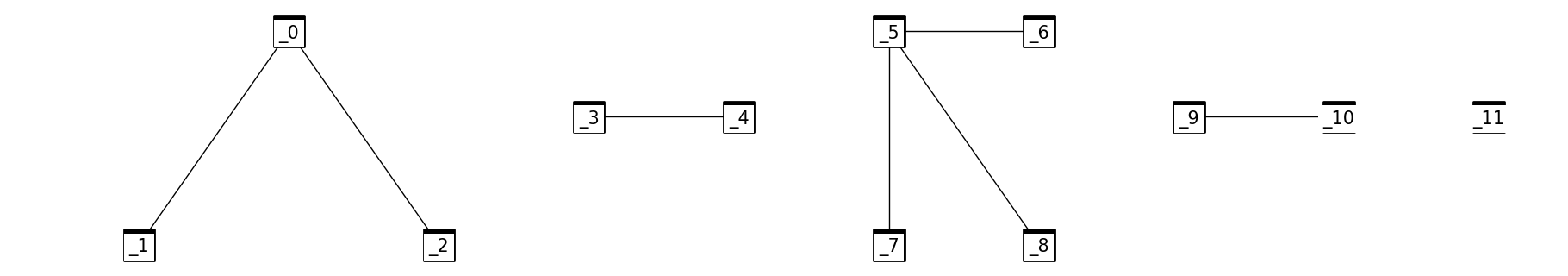For $$V[i], i = 0,1,2, ... , 11$$ $$$V[i] = [0, 1, 2, 3, 4, 5, 6, 7, 8, 9, 10, 11]$$$$Parent[i] = [-1, 0, 0, -1, 3, -1, 5, 5, 5, 10, -1, -1]$$$

So now we have a forest $$F$$ from the set of vertices $$S$$ and in $$F$$/$$S$$ we have multiple trees/disjoint sets…

### Find Algorithm#

Given a vertex $$i$$ in a certain tree/disjoint set, we want to know the root of that tree.

FIND(Parent[0:n-1], i, r) returns The root (r) of disjoint set that contains V[i]
rV[i]

while Parent[r] >= 0 do

r ← Parent[r]

endwhile

return r

### Minimum Spanning Tree with Kruskal’s algorithm#

KRUSKAL_MST(graph) returns a set MST contains edges of the tree
Forest ← {}
Size ← 0
for i ← 0 to n-1 do
Parent[i] ← -1
endfor
j ← 0
while Size <= n-1 and j < m do # m is the number of edges of set E = {ei = uivi | i in {1,…,m}}
jj + 1
FIND(Parent[0:n-1],uj,r)
FIND(Parent[0:n-1],vj,s)
if rs then
ForestForest ∐ {ej}
SizeSize + 1
UNION(Parent [0:n-1],r,s)
endifendwhileMSTForest
return MST

Let’s find the MST for all nodes in our search space (surrounding the University of Toronto). Here are some things to keep in mind:

• The graph may seem like a one big connected component but it actually isn’t. There are one-way streets which seem to connect the adjacent nodes on the graph, but in reality they are not connected (you can go from A to B but not in reverse).

• This will result in multiple MST at the end of the algorithm, one for each connected component.

• There may appear to loops in the MST, which is a major violation of tree-like structures. Recall however that what may seem like loops may just be one-way streets.

import networkx
import osmnx
from matplotlib.collections import LineCollection
import matplotlib.pyplot as plt
from smart_mobility_utilities.problem import *
from utils import *
from queue import PriorityQueue

reference = (43.661667, -79.395)
G = osmnx.graph_from_point(reference, dist=300, clean_periphery=True, simplify=True)
fig, ax = osmnx.plot_graph(G)

---------------------------------------------------------------------------
ModuleNotFoundError                       Traceback (most recent call last)
Input In , in <cell line: 5>()
3 from matplotlib.collections import LineCollection
4 import matplotlib.pyplot as plt
----> 5 from smart_mobility_utilities.problem import *
6 from utils import *
7 from queue import PriorityQueue

ModuleNotFoundError: No module named 'smart_mobility_utilities'

# Collect x,y coordinates for each node (for visualization purposes later)
node_Xs = [float(x) for _, x in G.nodes(data='x')]
node_Ys = [float(y) for _, y in G.nodes(data='y')]

Edges = PriorityQueue()

for source, destination, data in G.edges(keys=False, data=True):
Edges.put((data['length'],source,destination))

# Disjoint set to manage loops
nodes_set = {}
for i in Edges.queue:
nodes_set[i] = -1
nodes_set[i] = -1

# Returns -1 if the node has not been attached to another node during this run
def find_parent(node):
r = node
while nodes_set[r] >=0:
r = nodes_set[r]
return r

# The algorithm itself:
Forest = set()
Size = 0
j = 0
m = len(Edges.queue)
n = len(G.nodes)
while Size < n and j < m:
j += 1
# the shortest edge till this point
edge = Edges.queue.pop()
node1 = edge
node2 = edge
parent1 = find_parent(node1)
parent2 = find_parent(node2)
if parent1 != parent2:
Size += 1
nodes_set[node1] = node2

# Visualization
sources = [edge for edge in Forest]
destinations = [edge for edge in Forest]

fig, ax =  plt.subplots(figsize=(15, 11))
ax.set_facecolor('w')
lines = []
colorS = []
widthS = []
for u, v, data in G.edges(keys=False, data=True):
if 'geometry' in data:
xs, ys = data['geometry'].xy
lines.append(list(zip(xs, ys)))
else:
x1 = G.nodes[u]['x']
y1 = G.nodes[u]['y']
x2 = G.nodes[v]['x']
y2 = G.nodes[v]['y']
line = [(x1, y1), (x2, y2)]
lines.append(line)
if u in sources and v in destinations:
colorS.append('b')
widthS.append(2.5)
continue
if v in sources and u in destinations:
colorS.append('b')
widthS.append(2.5)
continue
colorS.append('r')
widthS.append(0.4)
lc = LineCollection(lines, colors=colorS, linewidths=widthS, alpha=0.3)
scat = ax.scatter(node_Xs, node_Ys,c='k', s=5)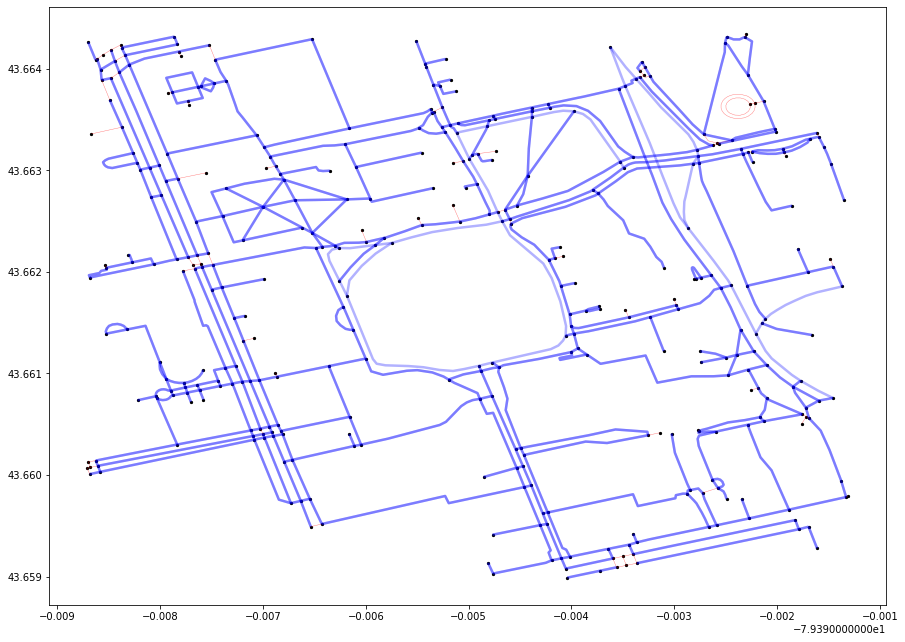## Hill Climbing#

The idea of the algorithm is quite simple:

Starting with a known (non-optimized) solution to a function, the algorithm checks the neighbours of that solution, and chooses the neighbour that is “more” optimized. The process is repeated until no “better” solution can be found, at which point the algorithm terminates.

While the algorithm works relatively well with convex problems, functions with multiple local maxima will often result in an answer that is not the global maximum. It also performs poorly when there are plateaus (a local set of solutions that are all similarly optimized).

HILL-CLIMBING(source,destination) return a route
current ← random route from source to destination
neighbours ← children of current

while min(neighbours) < current do
current ← min(neighbours)
neighbours ← children of current
return current

Here, we introduce a few new ideas.

First, we treat the route between two nodes as a function, the value of which is the distance between the two nodes. Second, we generate “children” of this function.

### The function#

We need to define a function $$f$$ that is our target for optimization.

$$f(x)$$ gives us the length of a route for some given route $$x \in Y$$, where $$Y$$ is the set of all possible routes between two specific nodes.

How do we generate $$x$$? We could just generate random permutations between the two nodes, filtering for permutations that are feasible, and optimize $$f$$ over these random, sparse permutations.

However, this method is not reproducible (because the permutations change every run).

Instead, we make a deterministic policy that generates a number of $$x \in Y$$ by successively “failing” nodes between the source and destination nodes. We then find the shortest path between the nodes before and after the “failed” nodes.

By failing the nodes in a deterministic fashion, we can say that we have a function and neighbourhood with defined size for a certain value so we can “rigorously” conduct a local search.

To generate our initial route and children routes, we will use the smart_mobility_utilities package. You can see how these routes are generated by consulting the documentation for that package.

# Setup the Graph, origin, and destination
from smart_mobility_utilities.common import Node
from smart_mobility_utilities.viz import draw_route

reference = (43.661667, -79.395)
G = osmnx.graph_from_point(reference, dist=300, clean_periphery=True, simplify=True)

origin = Node(graph=G, osmid=55808290)
destination = Node(graph=G, osmid=389677909)

from smart_mobility_utilities.common import randomized_search, cost
from smart_mobility_utilities.children import get_children
import matplotlib.pyplot as plt
from tqdm.notebook import tqdm

# Progress bar as this algorithm can take a long time
pbar = tqdm()
# Visualize the costs over time
costs = []
# Set the number of children to generate
num_children = 20

current = randomized_search(G, origin.osmid, destination.osmid)
costs.append(cost(G,current))
print("Initial cost:",costs)

neighbours = get_children(G,current,num_children=num_children)
shortest = min(neighbours , key = lambda route : cost(G, route))

print("Initial min(children):",cost(G,shortest))
while cost(G, shortest) < cost(G, current):
pbar.update(1)
current = shortest
neighbours = get_children(G,current,num_children=num_children)
shortest = min(neighbours , key = lambda route : cost(G, route))
costs.append(cost(G,current))
print(f"Current cost:",costs[-1],"|","min(children):",cost(G, shortest))

route = current

plt.xticks(range(len(costs)))
plt.title("Hill climbing: Shortest Path")
p = plt.plot(costs)
print("Final cost:",costs[-1])

Initial cost: 915.06
Initial min(children): 869.566
Current cost: 869.566 | min(children): 915.06
Final cost: 869.566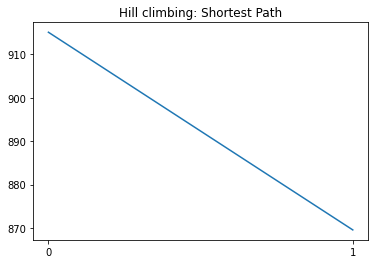draw_route(G,route)


While the implementation above is deterministic in nature, the initial route is still randomized. That means that it’s possible to get different results across runs.

Hill climbing will generally return some decent results as there are few local optimal points in the route function. However, with larger search spaces that will naturally have more local maxima and plateaus, it will get stuck fairly quickly.

## Hierarchical Approaches#

When facing routing problems at larger scales, such as those involving entire continents or graphs with millions of nodes, it is simply implausible to use basic approaches like Dijkstra or DFS. Instead, routing algorithms “prune” the search space in order to simplify the routing problem. Additionally, routing services may choose to precompute certain routes and cache them on servers, so that response times to user queries are reasonable.

Hierarchical search algorithms prune the search space by generating admissible heuristics that abstract the search space. Read more about the general approach of hierarchical methods at Faster Optimal and Suboptimal Hierarchical Search.

In this section, we’ll give a brief overview of two hierarchical approaches that aim to solve the shortest path problem, and show how their heuristics are computed. There will also be a python implementation of the Contraction Hierarchies example.

### Highway Hierarchies#

In this algorithm, the hierarchy “level” of each road/arc in the graph is calculated. This distinguishes the type of road segment (i.e. residential, national roads, highways). This is further supplemented by relevant data such as maximum designated driving speed, as well as number of turns in the road. After the heuristics are generated for the graph, the data is passed through a modified search function (bi-directional Dijkstra, A*, etc) that considers the distance to the destination and the potential expansion node class.

For example, the algorithm will generally consider highways as viable expansion nodes when it is still relatively further away from the target, and then will start to include national roads, and finally residential streets as it nears the destination.

While this approach “makes sense”, there are some disadvantages. First, the algorithm largely overlooks what kind of roads humans “prefer” to drive on. That is to say, which a highway might make sense for a given route, the user may “prefer” to take local roads (i.e. driving to a friend’s house who lives nearby). Secondly, highway hierarchies do not take into account factors such as traffic, which fluctuates often and adds significant cost to an “optimal” route.

### Contraction Hierarchies#

While the highway hierarchies algorithm may be useful for speeding up shortest path searches, it only considers three levels of road hierarchies. On the other hand, the contraction hierarchies algorithm introduced by Contraction Hierarchies: Faster and Simpler Hierarchical Routing in Road Networks has the same number of hierarchies as nodes in the graph, which is beneficial as increased number of hierarchies results in more pruning of the search space.

#### Contraction#

Taking any node $$V$$ from a graph $$G$$, remove $$V$$ as well as any connected edges.
Add any number of edges to $$G$$ such that the shortest distance for any pair of neighbouring nodes remains the same, even after $$V$$ has been removed. Below is an example: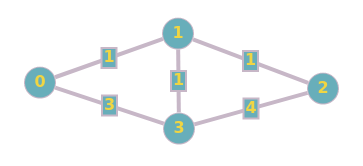Suppose we are contracting Node 1. By removing the node, we have now changed the shortest path for 0→2, 0→3, and 2→3. We can restore it by creating new edges. After the creation of new edges, we then reinsert any removed nodes and edges. We then update that node with its hierarchy level (which is just the order of contraction, from $$1$$ to $$n$$). See the below updated graph: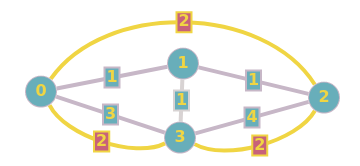This process is then repeated for all the other nodes. In our example, no other nodes need to be contracted, as removing any one of the remaining nodes does not affect the rest of the graph in terms of shortest path. This process will form shortcuts, which allow us to search the graph much faster, as we can ignore certain nodes that have been “pruned”.

#### Contraction Order#

Any order for node contraction will result in a successful algorithm, however some contraction ordering systems minimizes the number of new edges added to the graph, and thus the overall running time.

To utilize this, we can employ the idea of edge difference (ED). The ED of a node is $$S-E$$, where $$S$$ is the number of new edges added if that node were to be contracted, and $$E$$ is the number of edges that would be deleted. Minimizing ED is equivalent to minimizing the number of new edges added to the graph, and thus improves processing time.

### Example #1: Bi-directional Dijkstra with contraction#

After contracting a graph, we can run a bi-directional Dijkstra to compute all the shortest paths in the graph. Following this, any queries for the shortest path between any two nodes in the graph can be solved using a cached result.

There are some restrictions for our modified Dijkstra’s:

1. The forward expansion only considers arcs $$u,v$$, where $$level(u) > level(v)$$. This forms the upward graph, where only nodes with a higher contraction order can be relaxed.

2. The backward expansion only considers arcs $$u,v$$, where $$level(u) < level(v)$$. This forms the downward graph, where only nodes with a lower contraction order can be relaxed.

These restrictions help “prune” the search space and speed up the processing.

At the end, multiple paths are returned from source to destination, of which the shortest is selected to be the solution.

Let’s try this with the sample graph below:

import networkx
import math

G = networkx.Graph()

# Computing each contraction level requires a new graph, which is costly in terms of memory.
# To avoid this, we use a flag on every node to show its contraction state.

for i in range(1,15):

# Define the edges
edges = [
(1,2,{'weight':1}),
(1,3,{'weight':4}),
(2,3,{'weight':5}),
(2,4,{'weight':2}),
(3,4,{'weight':2}),
(3,7,{'weight':2}),
(3,8,{'weight':1}),
(3,9,{'weight':1}),
(4,5,{'weight':5}),
(5,10,{'weight':7}),
(6,7,{'weight':4}),
(6,8,{'weight':3}),
(6,10,{'weight':3}),
(6,5,{'weight':3}),
(6,9,{'weight':1}),
(7,8,{'weight':6}),
(8,9,{'weight':3}),
(8,13,{'weight':5}),
(9,12,{'weight':1}),
(9,10,{'weight':3}),
(10,11,{'weight':4}),
(11,12,{'weight':3}),
(11,13,{'weight':4}),
(12,13,{'weight':2}),
(14,1,{'weight':3}),
(14,13,{'weight':2})
]
# Add the edges to the graph and visualize it.
networkx.draw(G,with_labels=True)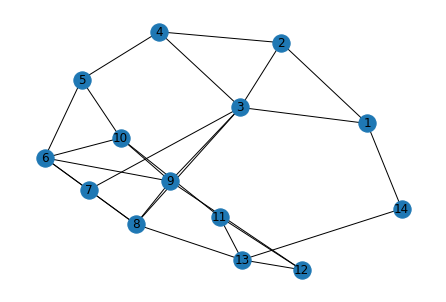Note

For this implementation we will be using two helper functions from smart_mobility_utilities.contraction.

The first is dijkstra_with_contraction, which simple runs a Dijkstra search on a graph, with the condition that the expansion nodes cannot be already contracted.

The second is calculate_edge_difference, which calculates the ED for every node in a given graph.

See the API docs for smart_mobility_utilities.contraction for more details.

We will first need to obtain the ED for the input graph:

def dijkstra_with_contraction(G, source, destination, contracted = None):
networkx.set_node_attributes(G, {contracted: True}, 'contracted')

shortest_path = dict()
heap = list()

for i in G.nodes():
if not networkx.get_node_attributes(G, 'contracted')[i]:
shortest_path[i] = math.inf
heap.append(i)
shortest_path[source] = 0

while len(heap) > 0:

q = min(heap, key = lambda node : shortest_path[node])
if q == destination:
networkx.set_node_attributes(G, {contracted: False}, 'contracted')
return shortest_path[q]
heap.remove(q)

for v in G[q]:
# if the node is contracted we skip it
if not networkx.get_node_attributes(G, 'contracted')[v]:
distance = shortest_path[q] + G[q][v]['weight']
if distance < shortest_path[v]:
shortest_path[v] = distance

networkx.set_node_attributes(G, {contracted: False}, 'contracted')

return math.inf # if we can't reach the destination

shortest_paths = dict()
for i in G.nodes():
shortest_paths[i] = dict()
for j in G.nodes():
shortest_paths[i][j] = dijkstra_with_contraction(G, i, j)

def calculate_edge_difference(G, shortest_paths):
edge_difference = list()
seenBefore = list()

for i in G.nodes():
# used in edge difference calculations
edges_incident = len(G[i])

# we will be deleting the node entry
# from the original shortest paths
# dictionary so we need to save its state
# for later iterations
contracted_node_paths = shortest_paths[i]
del shortest_paths[i]

# excluding the node that we have just contracted
new_graph = [*G.nodes()]
new_graph.remove(i)

# let's compute the new shortest paths between
# the nodes of the graph without the contracted
# node so we can see the changes and add arcs
# to the graph accordingly but that is in
# the algorithm itself
new_shortest_paths = dict()

for source in new_graph:
new_shortest_paths[source] = dict()
for destination in new_graph:
# path the contracted node "i" to compute new shortest paths accordingly
new_shortest_paths[source][destination] = dijkstra_with_contraction(G, \
source, \
destination, \
contracted = i)
# the add arcs to keep the graph all pairs shortest paths invariant
shortcuts = 0

for source in new_shortest_paths:
# we get a copy from the original and the new shortest paths dictionary
SP_contracted = new_shortest_paths[source]
SP_original = shortest_paths[source]
for destination in SP_contracted:
# this is statement so we don't add 2 arcs
# for the same pair of nodes
if [source, destination] in seenBefore: continue
seenBefore.append(sorted((source,destination)))

# if there is a difference between the original SP and
# post-contraction SP -- just add new arc
if SP_contracted[destination] != SP_original[destination]:
shortcuts += 1

# let's leave the dictionary as we took it
# from the last iteration
shortest_paths[i] = contracted_node_paths

# this is the value of the contraction
# heuristic for that node
ED = shortcuts - edges_incident
edge_difference.append((i, ED))
return edge_difference

edge_difference = calculate_edge_difference(G,shortest_paths)
edge_difference.sort(key=lambda pair: pair)

edge_difference

[(8, -5),
(10, -4),
(5, -3),
(7, -3),
(11, -3),
(14, 0),
(2, 2),
(1, 3),
(6, 4),
(13, 5),
(4, 7),
(12, 18),
(3, 26),
(9, 33)]


While this is a good start to optimizing the node contraction order, it is by no means perfect. Notice that the ED values calculated above assume the node is the only node removed from the graph. Because we are successively removing every node in the graph, the ED list will potentially become inaccurate after even the first contraction.

Recall however that any arbitrary contraction order results in a successful algorithm. While there are ED heuristics that are able to update the ED list after each contraction, each of them come with their own costs and benefits.

For the purposes of this example, our ED list will be sufficient.

# to keep track of the edges added after the algorithm finishes
edges_before = [*G.edges()]
current_graph = [*G.nodes()]

for node_ED in edge_difference:
node = node_ED

# now we will contract the given node through all iterations
networkx.set_node_attributes(G, {node: True}, 'contracted')

new_graph = current_graph
new_graph.remove(node)
current_shortest_paths = dict()
for source in new_graph:
current_shortest_paths[source] = dict()
for destination in new_graph:
current_shortest_paths[source][destination] = dijkstra_with_contraction(G,source, destination)

for source in current_shortest_paths:
SP_contracted = current_shortest_paths[source]
SP_original = shortest_paths[source]
for destination in SP_contracted:
if source == destination: continue
if SP_contracted[destination] != SP_original[destination]:
print("Added edge between ", source, destination," after contracting", node)

current_graph = new_graph

edges_after = [*G.edges()]
print("# edges before", len(edges_before))
print("# edges after", len(edges_after))

Added edge between  1 13  after contracting 14
Added edge between  2 13  after contracting 14
Added edge between  13 1  after contracting 14
Added edge between  13 2  after contracting 14
Added edge between  1 4  after contracting 2
Added edge between  4 1  after contracting 2
# edges before 26
# edges after 29


While it may seem like edges are being added twice, this is a simple graph, so adding an edge (b,a) when there is already an edge (a,b) has no effect.

# Visualize the newly created "shortcute" on the graph

# let's color these edges and draw the graph again
colors = ['r' if edge in added_edges else 'k' for edge in G.edges()]
widths = [2 if edge in added_edges else 1 for edge in G.edges()]
networkx.draw_networkx(G,with_labels=True,width=widths, edge_color=colors)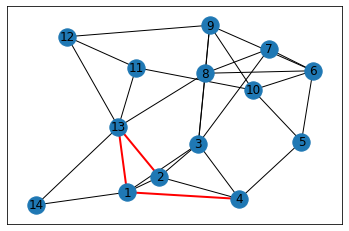# Reformat ED list to hierarchy for search purposes
hierarchical_order = dict()
for order, node in enumerate(edge_difference):
hierarchical_order[node] = order

hierarchical_order

{8: 0,
10: 1,
5: 2,
7: 3,
11: 4,
14: 5,
2: 6,
1: 7,
6: 8,
13: 9,
4: 10,
12: 11,
3: 12,
9: 13}


Let’s now find the shortest path from $$8$$ to $$12$$, using Dijkstra’s with contraction hierarchy.

source = 8
destination = 12

# Generating the upward graph

# initializing
SP_s = dict()
parent_s = dict()
unrelaxed_s = list()
for node in G.nodes():
SP_s[node] = math.inf
parent_s[node] = None
unrelaxed_s.append(node)
SP_s[source] = 0

# dijkstra
while unrelaxed_s:
node = min(unrelaxed_s, key = lambda node : SP_s[node])
unrelaxed_s.remove(node)
if SP_s[node] == math.inf: break
for child in G[node]:
# skip unqualified edges
if hierarchical_order[child] < hierarchical_order[node]: continue
distance = SP_s[node] + G[node][child]['weight']
if distance < SP_s[child]:
SP_s[child] = distance
parent_s[child] = node

# Generating the downward graph

# initializing
SP_t = dict()
parent_t = dict()
unrelaxed_t = list()
for node in G.nodes():
SP_t[node] = math.inf
parent_t[node] = None
unrelaxed_t.append(node)
SP_t[destination] = 0

# dijkstra
while unrelaxed_t:
node = min(unrelaxed_t, key = lambda node : SP_t[node])
unrelaxed_t.remove(node)
if SP_t[node] == math.inf: break
for child in G[node]:
# skip unqualified edges
if hierarchical_order[child] < hierarchical_order[node]: continue
distance = SP_t[node] + G[node][child]['weight']
if distance < SP_t[child]:
SP_t[child] = distance
parent_t[child] = node


With these, we now merge the common settled nodes from SP_d and SP_s, and find the minimum sum of values, This is our shortest path: the collision between the forward and backward expansions.

minimum = math.inf
merge_node = None
for i in SP_s:
if SP_t[i] == math.inf: continue
if SP_t[i] + SP_s[i] < minimum:
minimum = SP_t[i] + SP_s[i]
merge_node = i

print("Minimum:",minimum)
print("Merge node:",merge_node)

Minimum: 3
Merge node: 9

# Generate the route by getting the route from source to merge node, and from destination to merge node.
def route_dijkstra(parent, node):
route = []
while node != None:
route.append(node)
node = parent[node]
return route[::-1]
route_from_target = route_dijkstra(parent_t, merge_node)
route_from_source = route_dijkstra(parent_s, merge_node)
route = route_from_source + route_from_target[::-1][1:]
route

[8, 3, 9, 12]


Let’s compare this to networkx’s built in solver:

from networkx.algorithms.shortest_paths.weighted import single_source_dijkstra
single_source_dijkstra(G,source, destination)

(3, [8, 3, 9, 12])


#### Pruning#

We can actually check to see how many nodes were “pruned” from our graph, using the upward and downward graphs generated earlier.

unvisited = 0
for s_node, s_dist in SP_s.items():
for t_node, t_dist in SP_t.items():
if s_node == t_node and s_dist == t_dist == math.inf:
unvisited += 1
print(f"""Skipped {unvisited} nodes from a graph with {len(G)} total nodes,
resulting in pruning {unvisited/len(G)*100}% of the nodes in our search space.""")

Skipped 7 nodes from a graph with 14 total nodes,
resulting in pruning 50.0% of the nodes in our search space.


### Example #2: Equestrian Statue to Bahen Centre using Contraction Hierarchy#

We can also use the same method to calculate the shortest path between our statue and lecture hall.

Contraction hierarchies typically require incredibly powerful processing resources to run, especially when dealing with larger scales. Our ED function runs in $$O(n^2)$$ time, so for a graph of size 1,052 (our University of Toronto map), this requires 1,106,704 runs of dijkstra_with_contraction, which itself takes ~0.1 s per call. This would mean just calculating the ED would take 31 hours!

In order to make our example run in a more reasonable amount of time, we are going to reduce the complexity of the search space. Consider the following “simplified” road network:

import osmnx
import networkx as nx
from smart_mobility_utilities.viz import draw_route

reference = (43.661667, -79.395)

G = osmnx.graph_from_point(reference, dist=300, clean_periphery=True, simplify=True, network_type='drive_service')

fig, ax = osmnx.plot_graph(G)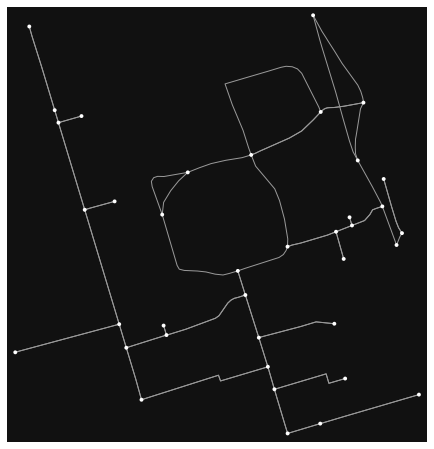This graph only contains 38 nodes and 73 edges, which is much more manageable. We can further remove any self-loops and parallel edges.

# Step 1: Remove Parallels and Loops, because Dijkstra doesn't like that
clean_G = nx.DiGraph()
clean_G.clear_edges()
for u in G.nodes:
for v in G[u]:
if u == v: G.remove_edge(u,v); continue
if len(G[u][v]) > 1:
keep = min(G[u][v], key=lambda x:G[u][v][x]['length'])
keep = G[u][v][keep]
continue

print(G)
G = clean_G
print(G)

MultiDiGraph with 38 nodes and 73 edges
DiGraph with 38 nodes and 71 edges

# Using functions from smart_mobility_utilities, we can generate shortest paths and edge differences very quickly
from smart_mobility_utilities.contraction import *
sp = shortest_paths(G)
ed = edge_differences(G,sp)

edges_before = [*G.edges()]
contract_graph(G,ed,sp) # Contract the graph according to ED

edges_after = [*G.edges()]

# Generate a hierarchy
hierarchical_order = dict()
for order, node in enumerate(ed):
hierarchical_order[node] = order

Added edges: 768

# Define the start and end
start = 36603405
end = 24959560

# Generate the upward and downward graphs
up, up_SP = generate_dijkstra(G,start,hierarchical_order)
down, down_SP = generate_dijkstra(G,end,hierarchical_order,'down')

minimum = math.inf
merge_node = None

for x in up_SP:
if x == start or x == end: continue
if up_SP[x] == math.inf or down_SP[x] == math.inf: continue
total = up_SP[x] + down_SP[x]
if total < minimum:
minimum = total
merge_node = x

route1 = build_route(G,start,merge_node,up)
route2 = build_route(G,end,merge_node,down)
route = route1[:-1] + route2[::-1]
print(route)
print("Cost:", round(minimum,3))

[36603405, 24960090, 24960068, 24960070, 249991437, 24960080, 5098988924, 854322047, 24959560]
Cost: 848.715


Once again, we can check to see how much the search space was pruned.

unvisited = 0
for s_node, s_dist in up_SP.items():
for t_node, t_dist in down_SP.items():
if s_node == t_node and s_dist == t_dist == math.inf:
unvisited += 1
print(f"""Skipped {unvisited} nodes from a graph with {len(G)} total nodes,
resulting in pruning {round(unvisited/len(G)*100,3)}% of the nodes in our search space.""")

Skipped 2 nodes from a graph with 38 total nodes,
resulting in pruning 5.263% of the nodes in our search space.

# Reset the Graph
G = osmnx.graph_from_point(reference, dist=300, clean_periphery=True, simplify=True, network_type='drive_service')
draw_route(G,route)


While this may not look very impressive, the gains from contraction hierarchies are much more pronounced with larger graphs. Typically, larger graphs will entail more impactful contractions that significantly increases the number of pruned nodes. Contraction hierarchies allow larger graphs to run costly algorithms like Dijkstra with a negative space cost. Consider the following:

• A regular Dijkstra search will need to maintain, with worst-case performance, a list of all traversed nodes and edges. This means a space complexity of $$O(bd)$$.

• A bidirectional implementation of Dijkstra will require a space complexity of $$O(bd/2) + O(bd/2)$$, but has the same time complexity (unless both expansions are run simultaneously, but that has its own complications).

• A contracted bidirectional Dijkstra will improve on both time and space costs, as the search space itself is modified.